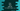# C++ program to find the sum of 1 + 1/2 + 1/3 + 1/4+....n## C++ program to find the sum of 1 + 1/2 + 1/3 + 1/4+…n:

In this C++ programming tutorial, we will learn how to find the sum of the series 1 + 1/2 + 1/3 + 1/4 + … upto nth term. This program will take the value of n as an input from the user and it will print the sum.

With this program, you will learn how to read user inputs, how to use loop and how to do simple mathematical calculations in C++.

### Algorithm to solve this problem:

If you look closely to the series 1 + 1/2 + 1/3 + 1/4…, the series is actually is the sum of the inverse of 1, 2, 3, 4,… etc. So, we can use a loop to run from 1 to n and in each iteration, we can add its inverse to a sum variable.

This series is also called a Harmonic progression. Harmonic progression is a series, the element of which is the reverse of an arithmetic progression. For example, if n1, n2, n3, n4, n5… is an arithmetic progression, 1/n1, 1/n2, 1/n3… is a harmonic progression.

In our case, it is a Harmonic progression series for the arithmetic progression 1, 2, 3, 4, 5….

• Initialize one sum variable as 0 to hold the sum.
• Use a loop to iterate from i = 1 to i = n.
• In each iteration, add the value of 1/i to sum.
• Finally, print the sum.

## C++ program:

Below is the complete C++ program that finds the sum of the series 1 + 1/2 + 1/3 +… upto nth term:

``````#include <iostream>
using namespace std;

float findSum(int n)
{
float sum = 0;

for (float i = 1; i <= n; i++)
{
sum += 1 / i;
}

return sum;
}

int main()
{
int n;

cout
<< "Enter the value of n: "
<< endl;
cin >> n;

cout << "Sum: " << findSum(n) << endl;
}``````

Here,

• n is an integer variable to hold the value of n that is entered by the user.
• By using cin, we are reading the value of n.
• The value of sum is calculated by using the findSum method. This method takes the value of n as its parameter and returns the sum, which is a floating point value.

• In findSum, we have initialized one sum variable as 0, which is a float value.
• The for loop runs from i = 1 to i = n. Inside this loop, it is adding the value of 1/i to sum in each iteration.
• Once the loop ends, it returns sum, which holds the required sum.

### Sample output:

If you run the above program, it will print output as like below:

``````Enter the value of n:
4
Sum: 2.08333

Enter the value of n:
6
Sum: 2.45

Enter the value of n:
11
Sum: 3.01988``````